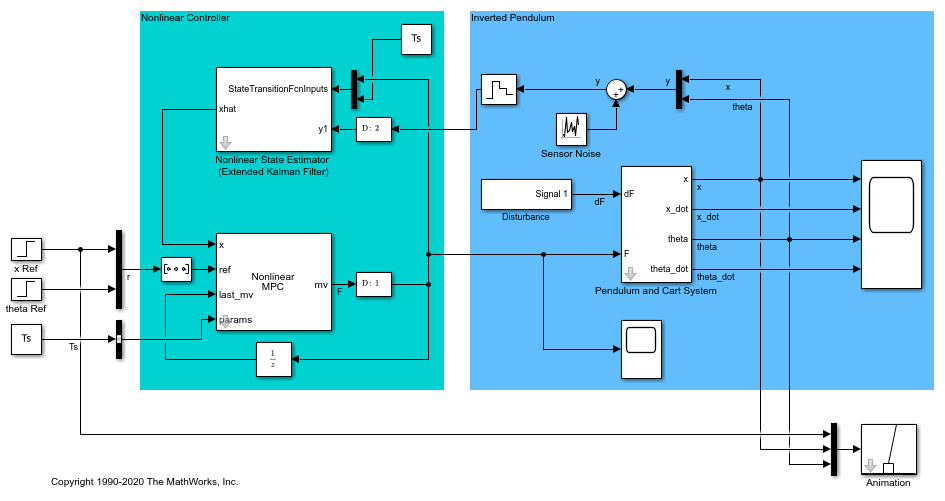# createParameterBus

Create Simulink bus object and configure Bus Creator block for passing model parameters to Nonlinear MPC Controller block

## Syntax

``createParameterBus(nlmpcobj,nlmpcblk,busName,parameters)``

## Description

example

````createParameterBus(nlmpcobj,nlmpcblk,busName,parameters)` creates a `Simulink.Bus` object, `busName`, in the MATLAB® workspace for passing model parameters to a Nonlinear MPC Controller block, `nlmpcblk`. `createParameterBus` requires you to connect a Bus Creator block to the Nonlinear MPC Controller block in advance so that it can configure the Bus Creator block to use the bus object.```

## Examples

collapse all

Create a nonlinear MPC controller with four states, two outputs, and one input.

```nlobj = nlmpc(4,2,1); ```
```In standard cost function, zero weights are applied by default to one or more OVs because there are fewer MVs than OVs. ```

Specify the sample time and horizons of the controller.

```Ts = 0.1; nlobj.Ts = Ts; nlobj.PredictionHorizon = 10; nlobj.ControlHorizon = 5; ```

Specify the state function for the controller, which is in the file `pendulumDT0.m`. This discrete-time model integrates the continuous-time model defined in `pendulumCT0.m` using a multistep forward Euler method.

```nlobj.Model.StateFcn = "pendulumDT0"; nlobj.Model.IsContinuousTime = false; ```

The prediction model uses an optional parameter, `Ts`, to represent the sample time. Specify the number of parameters.

```nlobj.Model.NumberOfParameters = 1; ```

Specify the output function of the model, passing the sample-time parameter as an input argument.

```nlobj.Model.OutputFcn = @(x,u,Ts) [x(1); x(3)]; ```

Define standard constraints for the controller.

```nlobj.Weights.OutputVariables = [3 3]; nlobj.Weights.ManipulatedVariablesRate = 0.1; nlobj.OV(1).Min = -10; nlobj.OV(1).Max = 10; nlobj.MV.Min = -100; nlobj.MV.Max = 100; ```

```mdl = 'mpc_pendcartNMPC'; open_system(mdl) ```In this model, the Nonlinear MPC Controller block is configured to use the controller `nlobj`.

To use the optional parameter in the prediction model, the model has a Simulink Bus block connected to the `params` input port of the Nonlinear MPC Controller block. To configure this bus block to use the `Ts` parameter, create a Bus object in the MATLAB® workspace, and configure the Bus Creator block to use this object. Name the Bus object `'myBusObject'`.

```createParameterBus(nlobj,[mdl '/Nonlinear MPC Controller'],'myBusObject',{Ts}); bdclose(mdl) ```
```A Simulink Bus object "myBusObject" created in the MATLAB Workspace, and Bus Creator block "mpc_pendcartNMPC/Nonlinear MPC Controller" is configured to use it. ```

## Input Arguments

collapse all

Nonlinear MPC controller, specified as an `nlmpc` object.

Block path of Nonlinear MPC Controller block, specified as a string or character vector.

Name of Simulink bus object to be created in the MATLAB workspace and set in the Bus Creator block, specified as a string or character vector.

The corresponding Bus Creator block must already be connected to the `params` input port of the Nonlinear MPC Controller block specified by `nlmpcblk`. Also, the Bus Creator block must have the correct number of input ports, and these ports must already be properly connected.

Nominal prediction model parameter values, specified as a cell array of length Np, where Np is equal to `nlmpcobj.Model.NumberOfParameters`. The order of the parameters must match the order specified in the model functions, and each parameter must be a numeric parameter with the correct dimensions.

## Version History

Introduced in R2018b